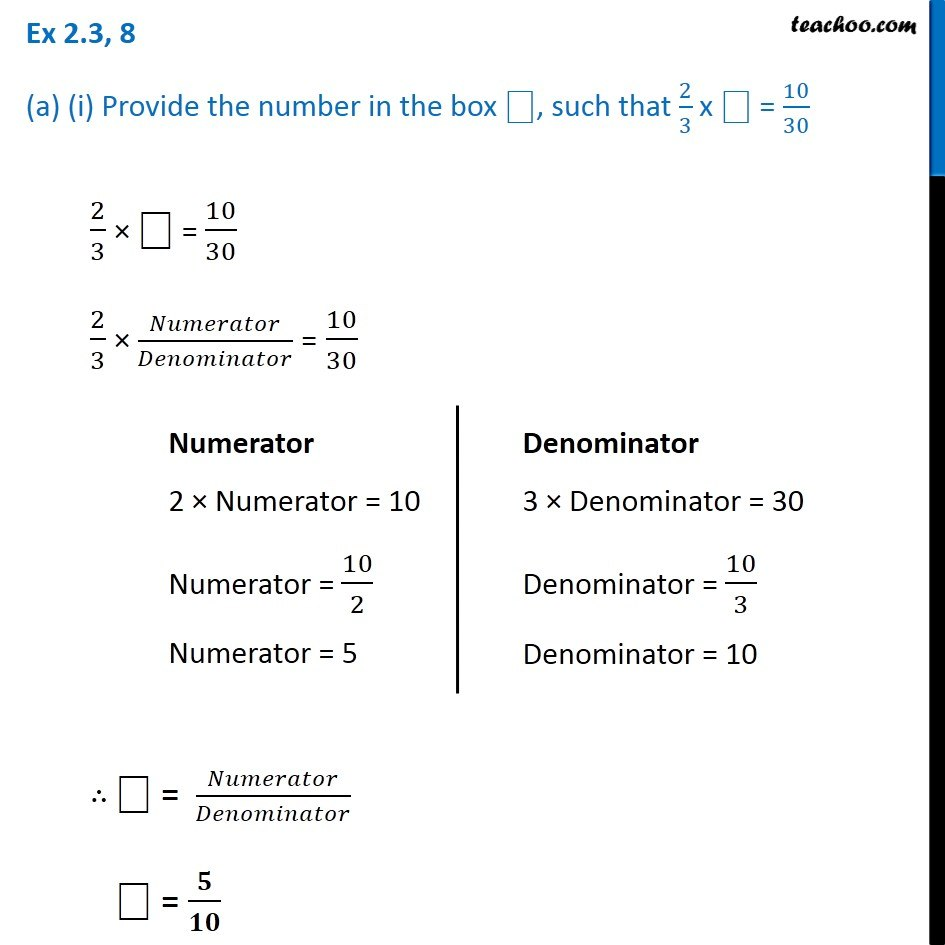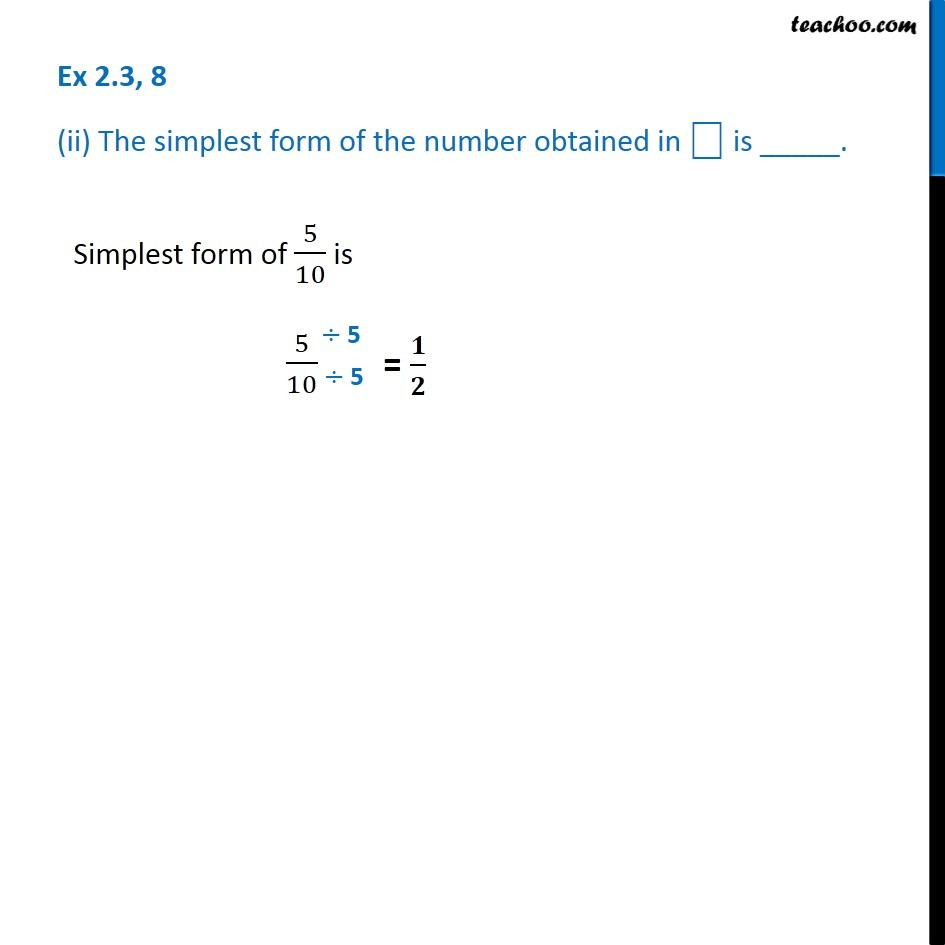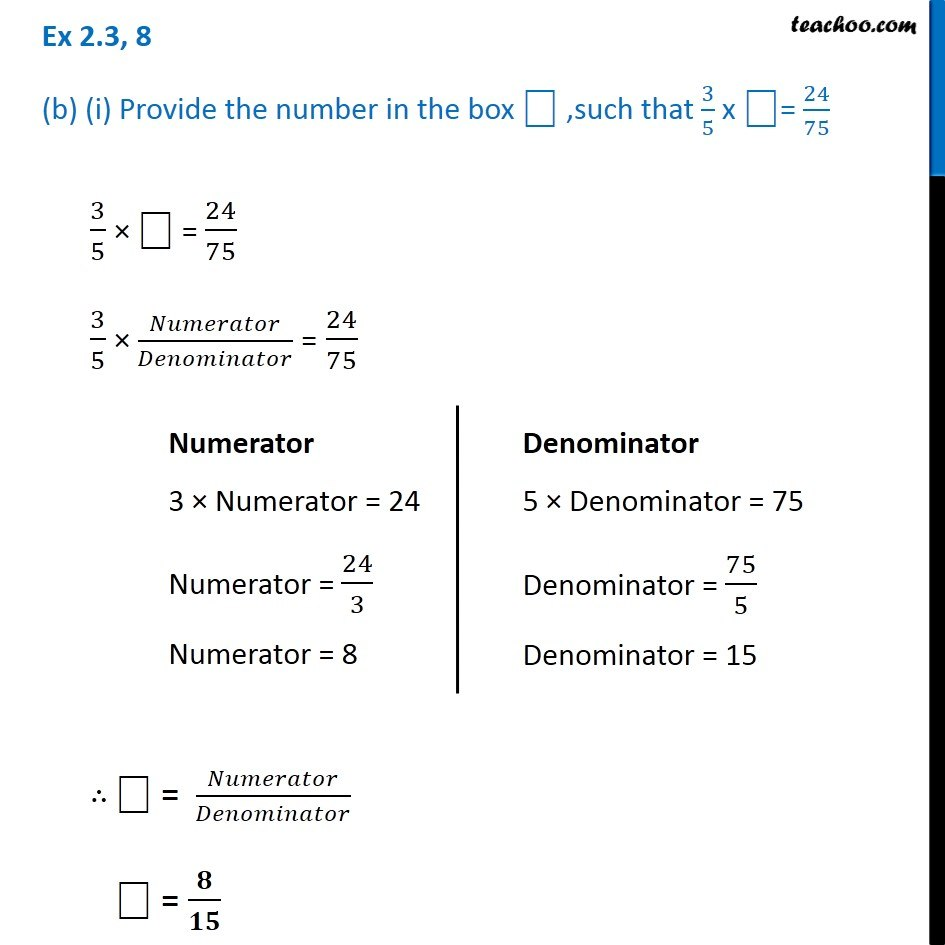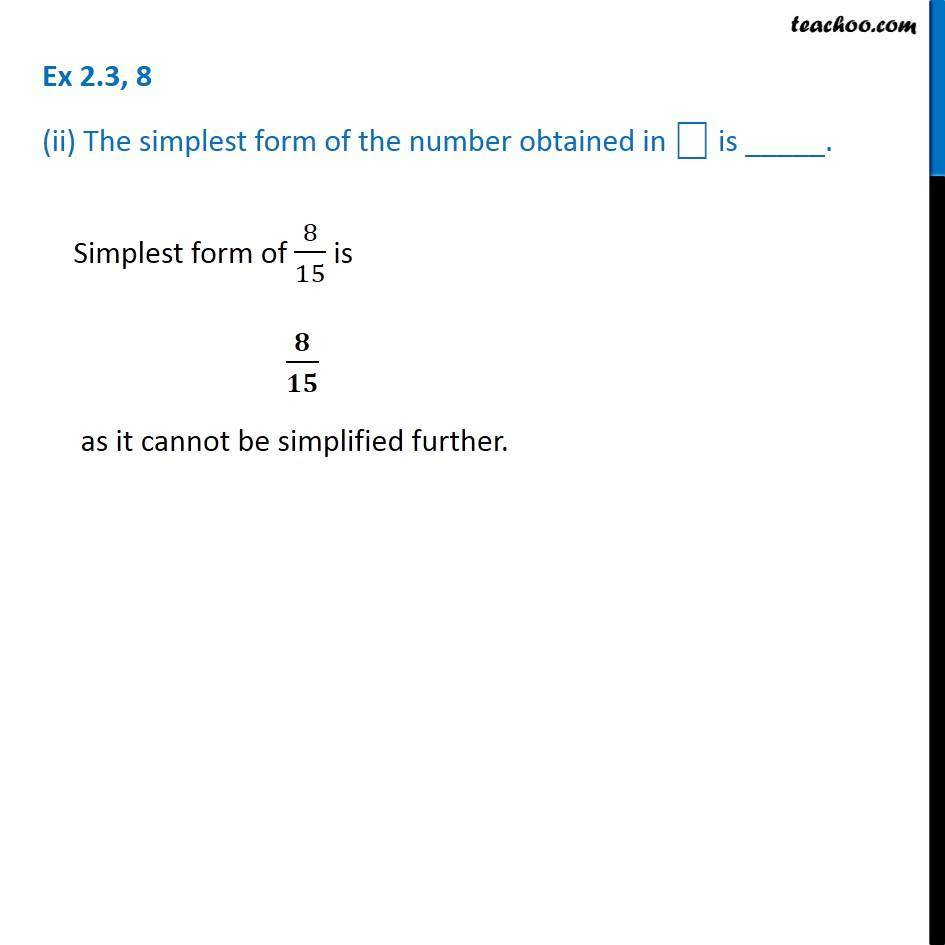Subscribe to our Youtube Channel - https://you.tube/teachoo

1. Chapter 2 Class 7 Fractions and Decimals
2. Concept wise
3. Multiplication of Fractions

Transcript

Ex 2.3, 8 (a) (i) Provide the number in the box ⎕, such that 2/3 x ⎕ = 10/302/3 × ⎕ = 10/30 2/3 × 𝑁𝑢𝑚𝑒𝑟𝑎𝑡𝑜𝑟/𝐷𝑒𝑛𝑜𝑚𝑖𝑛𝑎𝑡𝑜𝑟 = 10/30 ∴ ⎕ = 𝑁𝑢𝑚𝑒𝑟𝑎𝑡𝑜𝑟/𝐷𝑒𝑛𝑜𝑚𝑖𝑛𝑎𝑡𝑜𝑟 ⎕ = 𝟓/𝟏𝟎 Numerator 2 × Numerator = 10 Numerator = 10/2 Numerator = 5 Denominator 3 × Denominator = 30 Denominator = 10/3 Denominator = 10 Ex 2.3, 8 (ii) The simplest form of the number obtained in ⎕ is _____.Simplest form of 5/10 is 5/10 Ex 2.3, 8 (b) (i) Provide the number in the box ⎕ ,such that 3/5 x ⎕= 24/753/5 × ⎕ = 24/75 3/5 × 𝑁𝑢𝑚𝑒𝑟𝑎𝑡𝑜𝑟/𝐷𝑒𝑛𝑜𝑚𝑖𝑛𝑎𝑡𝑜𝑟 = 24/75 ∴ ⎕ = 𝑁𝑢𝑚𝑒𝑟𝑎𝑡𝑜𝑟/𝐷𝑒𝑛𝑜𝑚𝑖𝑛𝑎𝑡𝑜𝑟 ⎕ = 𝟖/𝟏𝟓 Numerator 3 × Numerator = 24 Numerator = 24/3 Numerator = 8 Denominator 5 × Denominator = 75 Denominator = 75/5 Denominator = 15 Ex 2.3, 8 (ii) The simplest form of the number obtained in ⎕ is _____.Simplest form of 8/15 is 𝟖/𝟏𝟓 as it cannot be simplified further.

Multiplication of Fractions